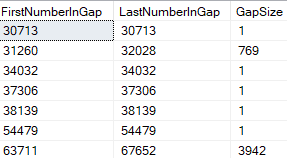# How to Find Gaps in a Number Column

Hi there,
My table has 2 columns (for example:
ID (its an identity column)
Name

I want to select all instances of gaps in the ID values in table Table1

For example, if these are no rows for ID 4 and 5, the query would select these rows
3 John
6 Mary

and for
7 Robert
8 Sue these would not be selected as there is no gap in ID.

Can this be done in TSQL?
Thanks again.

Please always provide sample data. Also you have not show us how you would like the final result to look like. Here is one of dozens of ways to do it. scrapped this from online.

``````;WITH Data(Datum)
AS
(
select 3 union
select 6
),
CTE
AS
(
SELECT MIN(Datum) Start,
MAX(Datum) Finish
FROM Data
UNION ALL
SELECT Start + 1,
Finish
FROM CTE
WHERE Start < Finish
)
SELECT *
FROM CTE
WHERE NOT EXISTS
(
SELECT 1 FROM Data WHERE Data.Datum = cte.Start
)
``````

another way.

``````declare @dbforever table(ID int, Name nvarchar(1500), SomeDate datetime )

insert into @dbforever(id, Name, SomeDate)

WITH SourceData AS
(
SELECT
ID AS ID,
RowNum = ROW_NUMBER() OVER (ORDER BY ID)
FROM @dbforever
),
Ranked AS
(
SELECT
*,
DENSE_RANK() OVER (ORDER BY ID - RowNum) As Series
FROM SourceData
),
Counted AS
(
SELECT
*,
COUNT(*) OVER (PARTITION BY Series) AS SCount
FROM Ranked
),
Gaps AS
(
SELECT
MinID = MIN(ID),
MaxID = MAX(ID),
Series
FROM
Counted
GROUP BY Series
)

SELECT
FirstNumberInGap = (a.MaxID + 1),
LastNumberInGap = (b.MinID - 1),
GapSize = ((b.MinID - 1) - a.MaxID)
FROM Gaps a
JOIN Gaps b ON a.Series + 1 = b.Series
ORDER BY FirstNumberInGap

``````
1 Like

Thank you, yosiaz. That helps but this is what I'm getting:
WITH SourceData AS
(
SELECT
ANBRID AS ANBRID,
RowNum = ROW_NUMBER() OVER (ORDER BY ANBRID)
FROM MyTable
),
Ranked AS
(
SELECT
*,
DENSE_RANK() OVER (ORDER BY ANBRID - RowNum) As Series
FROM SourceData
),
Counted AS
(
SELECT
,
COUNT(
) OVER (PARTITION BY Series) AS SCount
FROM Ranked
),
Gaps AS
(
SELECT
MinID = MIN(ANBRID),
MaxID = MAX(ANBRID),
Series
FROM
Counted
GROUP BY Series
)

SELECT
FirstNumberInGap = (a.MaxID + 1),
LastNumberInGap = (b.MinID - 1),
GapSize = ((b.MinID - 1) - a.MaxID)
FROM Gaps a
JOIN Gaps b ON a.Series + 1 = b.Series
ORDER BY FirstNumberInGap

1. It returns gaps yes but also some ranges where there is no gap (meaning the Gap Size is 1) as you see here:2. I want to also select another column name Parent and have Parent selected on every row that gets returned, Can't figure how to do that.
Thanks

Try this one. Also play around with it, to get it to do what you want. That is the fun part of learning new things. poke around. understand why.

``````;WITH Data(Datum)
AS
(
select 31260 union
select 32028 union
select 30713
),
CTE
AS
(
SELECT MIN(Datum) Start,
MAX(Datum) Finish
FROM Data
UNION ALL
SELECT Start + 1,
Finish
FROM CTE
WHERE Start < Finish
)
SELECT *
FROM CTE
WHERE NOT EXISTS
(
SELECT 1 FROM Data WHERE Data.Datum = cte.Start
)
OPTION(MAXRECURSION 0)
``````

So I used the top approach, inserting the results into a temp table. But the results were the middle of the gap. For example, if the range went from 2 to 4, the code returns 3. So I subtracted 1 and added 1 to get the Starting value of the range that contains a gap. Inserted those values into a table.
It's a Kinda dumbed down technique I admit but it worked.

1 Like